Over a million developers have joined DZone.{{announcement.body}}
{{announcement.title}}

# Foundations of Machine Learning: Part 4

DZone 's Guide to

# Foundations of Machine Learning: Part 4

### Explore penalization and variables selection.

· AI Zone ·
Free Resource

Comment (0)

Save
{{ articles.views | formatCount}} ViewsThis post is the eighth one of our series onthe history and foundations of econometric and machine learning models. The first four were on econometrics techniques. Part 7 is online here.

## Penalization and Variables Selection

One important concept in econometrics is Ockham's razor — also known as the law of parsimony (lex parsimoniae) — which can be related to abductive reasoning.

Akaike's criterion was based on a penalty of likelihood taking into account the complexity of the model (the number of explanatory variables retained). If in econometrics, it is customary to maximize the likelihood (to build an asymptotically unbiased estimator), and to judge the quality of the ex-post model by penalizing the likelihood, the strategy here will be to penalize ex-ante in the objective function, even if it means building a biased estimator. Typically, we will build: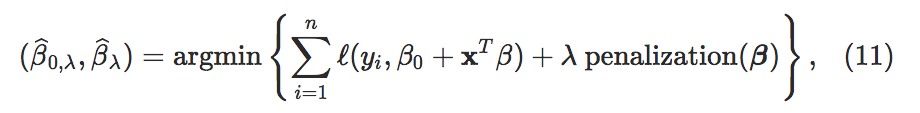where the penalty function will often be a norm ∥⋅∥ chosen a priori, and a penalty parameter λ (we find in a way the distinction between AIC and BIC if the penalty function is the complexity of the model — the number of explanatory variables retained). In the case of the ℓ2 norm, we find the ridge estimator, and for the 1 norm, we find the lasso estimator ("Least Absolute Shrinkage and Selection Operator"). The penalty previously used involved the number of degrees of freedom of the model, so it may seem surprising to use β∥ℓ2 as in the ridge regression. However, we can envisage a Bayesian vision of this penalty. It should be recalled that in a Bayesian model: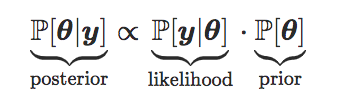or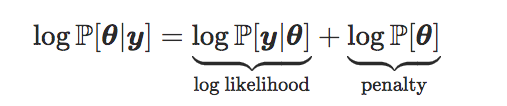In a Gaussian linear model, if we assume that the a priori law oftheta θ follows a centred Gaussian distribution, we find a penalty based on a quadratic form of the components of thetaθ.

Before going back in detail to these two estimators, obtained using the 1 or 2 norm, let us return for a moment to a very similar problem: the best choice of explanatory variables. Classically (and this will be even more true in large dimension), we can have a large number of explanatory variables, p, but many are just noise, in the sense that βj=0 for a large number of j. Let s be the number of (really) relevant covariates, s=#S, with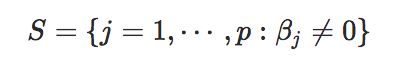If we note Xthe matrix composed of the relevant variables (in columns), then we assume that the real model is of the form
y=\mathbf{x}_S^T \beta_S+\varepsilon
y=xSTβS+ε
. Intuitively, an interesting estimator would then be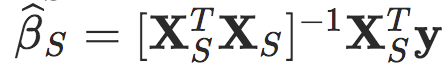but this estimator is only theoretical because the set S is unknown, here. This estimator can actually be seen as the oracle estimator mentioned above. One may then be tempted to solve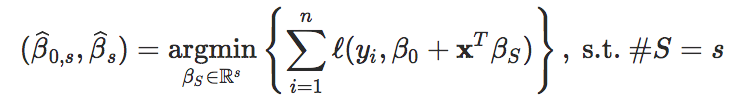This problem was introduced by Foster & George (1994) using the 0 notation. More precisely, let us define here the following three norms, where aR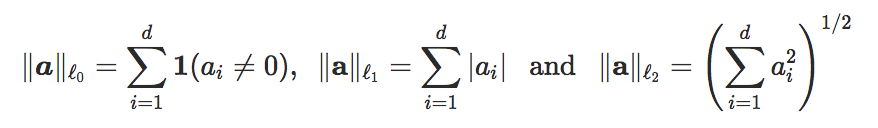Table 1: Constrained optimization and regularization.Let us consider the optimization problems in Table 1. If we consider the classical problem where the quadratic norm is used for , the two problems of the equation (ℓ1) of Table 1 are equivalent, in the sense that, for any solution (β⋆,s) to the left problem, there is λ such that
(\beta^\star,\lambda^\star)
(β⋆,λ⋆)
is the solution of the right problem; and vice versa. The result is also true for problems (ℓ2). These are indeed convex problems. On the other hand, the two problems (ℓ0) are not equivalent: if for (β⋆,λ⋆) solution of the right problem, there is s  such that β is solution of the left problem, the reverse is not true. More generally, if you want to use an p  norm, sparsity is obtained if p≤1 whereas you need p≥1 to have the convexity of the optimization program.

One may be tempted to resolve the penalized program (ℓ0) directly, as suggested by Foster & George (1994). Numerically, it is a complex combinatorial problem in large dimension (Natarajan (1995) notes that it is a NP-difficult problem), but it is possible to show that if  λσ2log(p), then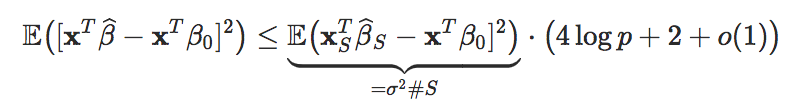Observe that in this case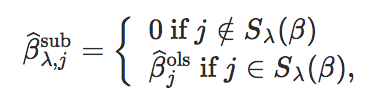where Sλ(β) refers to all non-zero coordinates when solving (ℓ0).

The problem (ℓ2) is strictly convex if  is the quadratic norm, in other words, the Ridge estimator is always well defined, with in addition an explicit form for the estimator,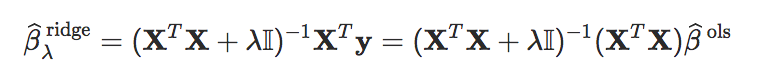Therefore, it can be deduced thatandWith a matrix of orthonormal explanatory variables (i.e. XTX=I), the expressions can be simplified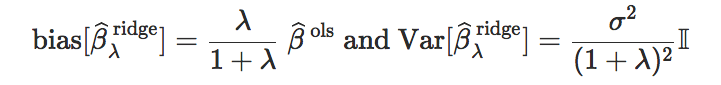Observe that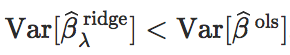And because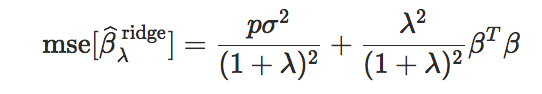we obtain an optimal value for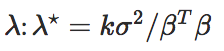On the other hand, ifell ℓ is no longer the quadratic norm but the 1 norm, the problem (ℓ1) is not always strictly convex, and in particular, the optimum is not always unique (for example if XTX is singular). But if it is strictly convex, then predictions Xβ will be unique. It should also be noted that two solutions are necessarily consistent in terms of sign of coefficients: it is not possible to have βj<0 for one solution and
\beta_j>0
βj>0
for another. From a heuristic point of view, the program (ℓ1) is interesting because it allows us to obtain, in many cases, a corner solution, which corresponds to a problem resolution of type (ℓ0) — as shown visually in Figure 2.Figure 2 : Penalization based on norms ℓ0ℓ1, and ℓ2 (from Hastie et al. (2016)).

Let us consider a very simple model:
y_i=x_i \beta+\varepsilon
yi=xiβ+ε
, with a penalty ℓ1 and a loss function 2. The problem (2) then becomesThe first order condition is then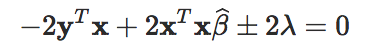And the sign of the last term depends on the sign of β. Suppose that the least square estimator (obtained by setting λ=0) is (strictly) positive, i. e. yTx>0. If λ is not too big, we can imagine that β is of the same sign as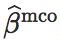and therefore, the condition becomesand the solution is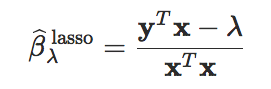By increasing λ, there will be a time such that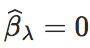If we increase λ a bit little more,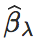does not become negative because, in this case, the last term of the first order condition changes, and we try to solve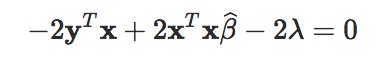whose solution is then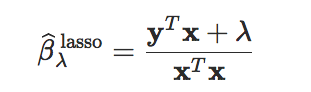But this solution is positive (we assumed yTx>0), and so it is possible to have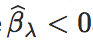at the same time. Also, after a while,which is then a corner solution. Things are of course more complicated in larger dimensions (Tibshirani & Wasserman (2016) goes back at length on the geometry of the solutions) but as Candès & Plan (2009) notes, under minimal assumptions guaranteeing that the predictors are not strongly correlated, the Lasso obtains a quadratic error almost as good as if we had an oracle providing perfect information on the set of
\beta_j
βj's that are not zero. With some additional technical hypotheses, it can be shown that this estimator is "sparsistant" in the sense that the support ofis that of β, in other words, Lasso has made it possible to select variables (more discussions on this point can be obtained in Hastie et al. (2016)).

More generally, it can be shown thatis a biased estimator, but may be of sufficiently low variance that the mean square error is lower than using least squares. To compare the three techniques, relative to the least square estimator (obtained when λ=0), if we assume that the explanatory variables are orthonormal, then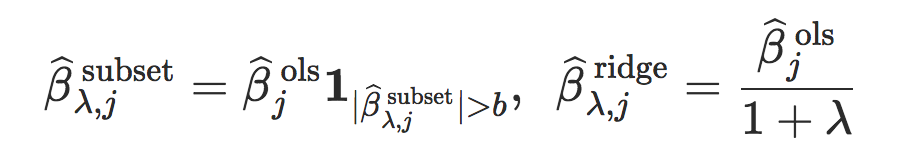and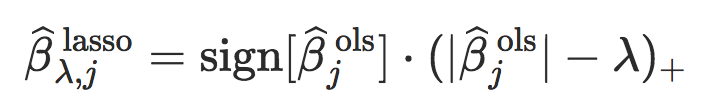Figure 3: Penalization based on norms (from Hastie et al. (2016)).

To be continued with probably a final post this week (references are online here)...
Topics:
artificial intelligence ,machine learning ,tutorial ,penalization ,variables selection ,econometrics

Comment (0)

Save
{{ articles.views | formatCount}} Views

Published at DZone with permission of

Opinions expressed by DZone contributors are their own.

# {{ parent.title || parent.header.title}}

{{ parent.tldr }}

{{ parent.urlSource.name }}